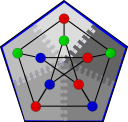#Boost C++ Libraries

...one of the most highly regarded and expertly designed C++ library projects in the world.

#Vertex List Graph Adaptor

```template<typename Graph, typename GlobalIndexMap>
{
public:
const GlobalIndexMap& index_map = GlobalIndexMap());
};

template<typename Graph, typename GlobalIndexMap>
make_vertex_list_adaptor(const Graph& g, const GlobalIndexMap& index_map);

template<typename Graph>
```

The vertex list graph adaptor adapts any model of Distributed Vertex List Graph in a Vertex List Graph. In the former type of graph, the set of vertices is distributed across the process group, so no process has access to all vertices. In the latter type of graph, however, every process has access to every vertex in the graph. This is required by some distributed algorithms, such as the implementations of Minimum spanning tree algorithms.

# Where Defined

The vertex_list_adaptor class template takes a Distributed Vertex List Graph and a mapping from vertex descriptors to global vertex indices, which must be in the range [0, n), where n is the number of vertices in the entire graph. The mapping is a Readable Property Map whose key type is a vertex descriptor.

On construction, the vertex list adaptor performs an all-gather operation to create a list of all vertices in the graph within each process. It is this list that is accessed via vertices and the length of this list that is accessed via num_vertices. Due to the all-gather operation, the creation of this adaptor is a collective operation.

These function templates construct a vertex list adaptor from a graph and, optionally, a property map that maps vertices to global index numbers.

## Parameters

IN: Graph& g
The graph type must be a model of Distributed Vertex List Graph.
IN: GlobalIndexMap index_map

A Distributed property map whose type must model Readable property map that maps from vertices to a global index. If provided, this map must be initialized prior to be passed to the vertex list adaptor.

Default: A property map of unspecified type constructed from a distributed iterator_property_map that uses the vertex_index property map of the underlying graph and a vector of vertices_size_type.

## Complexity

These operations require O(n) time, where n is the number of vertices in the graph, and O(n) communication per node in the BSP model.

Copyright (C) 2004 The Trustees of Indiana University.

Authors: Douglas Gregor and Andrew Lumsdaine